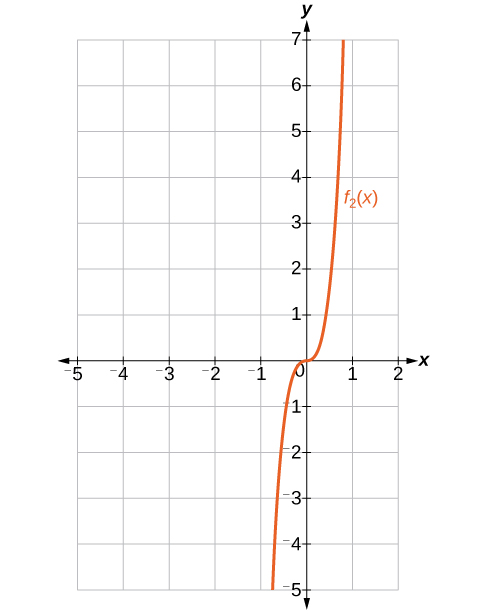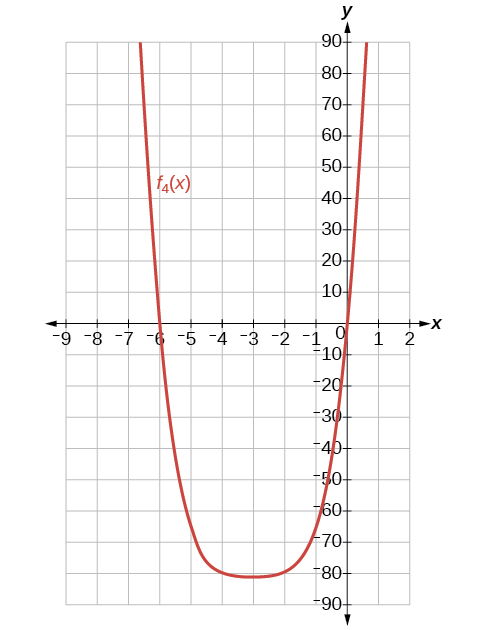# 13.6 Binomial theorem  (Page 3/6)

 Page 3 / 6

## Key equations

 Binomial Theorem ${\left(x+y\right)}^{n}=\sum _{k-0}^{n}\left(\begin{array}{c}n\\ k\end{array}\right){x}^{n-k}{y}^{k}$ $\left(r+1\right)th\text{\hspace{0.17em}}$ term of a binomial expansion $\left(\begin{array}{c}n\\ r\end{array}\right){x}^{n-r}{y}^{r}$

## Key concepts

• $\left(\begin{array}{c}n\\ r\end{array}\right)\text{\hspace{0.17em}}$ is called a binomial coefficient and is equal to $C\left(n,r\right).\text{\hspace{0.17em}}$ See [link] .
• The Binomial Theorem allows us to expand binomials without multiplying. See [link] .
• We can find a given term of a binomial expansion without fully expanding the binomial. See [link] .

## Verbal

What is a binomial coefficient, and how it is calculated?

A binomial coefficient is an alternative way of denoting the combination $\text{\hspace{0.17em}}C\left(n,r\right).\text{\hspace{0.17em}}$ It is defined as $\text{\hspace{0.17em}}\left(\begin{array}{c}n\\ r\end{array}\right)=\text{\hspace{0.17em}}C\left(n,r\right)\text{\hspace{0.17em}}=\frac{n!}{r!\left(n-r\right)!}.$

What role do binomial coefficients play in a binomial expansion? Are they restricted to any type of number?

What is the Binomial Theorem and what is its use?

The Binomial Theorem is defined as $\text{\hspace{0.17em}}{\left(x+y\right)}^{n}=\sum _{k=0}^{n}\left(\begin{array}{c}n\\ k\end{array}\right){x}^{n-k}{y}^{k}\text{\hspace{0.17em}}$ and can be used to expand any binomial.

When is it an advantage to use the Binomial Theorem? Explain.

## Algebraic

For the following exercises, evaluate the binomial coefficient.

$\left(\begin{array}{c}6\\ 2\end{array}\right)$

15

$\left(\begin{array}{c}5\\ 3\end{array}\right)$

$\left(\begin{array}{c}7\\ 4\end{array}\right)$

35

$\left(\begin{array}{c}9\\ 7\end{array}\right)$

$\left(\begin{array}{c}10\\ 9\end{array}\right)$

10

$\left(\begin{array}{c}25\\ 11\end{array}\right)$

$\left(\begin{array}{c}17\\ 6\end{array}\right)$

12,376

$\left(\begin{array}{c}200\\ 199\end{array}\right)$

For the following exercises, use the Binomial Theorem to expand each binomial.

${\left(4a-b\right)}^{3}$

$64{a}^{3}-48{a}^{2}b+12a{b}^{2}-{b}^{3}$

${\left(5a+2\right)}^{3}$

${\left(3a+2b\right)}^{3}$

$27{a}^{3}+54{a}^{2}b+36a{b}^{2}+8{b}^{3}$

${\left(2x+3y\right)}^{4}$

${\left(4x+2y\right)}^{5}$

$1024{x}^{5}+2560{x}^{4}y+2560{x}^{3}{y}^{2}+1280{x}^{2}{y}^{3}+320x{y}^{4}+32{y}^{5}$

${\left(3x-2y\right)}^{4}$

${\left(4x-3y\right)}^{5}$

$1024{x}^{5}-3840{x}^{4}y+5760{x}^{3}{y}^{2}-4320{x}^{2}{y}^{3}+1620x{y}^{4}-243{y}^{5}$

${\left(\frac{1}{x}+3y\right)}^{5}$

${\left({x}^{-1}+2{y}^{-1}\right)}^{4}$

$\frac{1}{{x}^{4}}+\frac{8}{{x}^{3}y}+\frac{24}{{x}^{2}{y}^{2}}+\frac{32}{x{y}^{3}}+\frac{16}{{y}^{4}}$

${\left(\sqrt{x}-\sqrt{y}\right)}^{5}$

For the following exercises, use the Binomial Theorem to write the first three terms of each binomial.

${\left(a+b\right)}^{17}$

${a}^{17}+17{a}^{16}b+136{a}^{15}{b}^{2}$

${\left(x-1\right)}^{18}$

${\left(a-2b\right)}^{15}$

${a}^{15}-30{a}^{14}b+420{a}^{13}{b}^{2}$

${\left(x-2y\right)}^{8}$

${\left(3a+b\right)}^{20}$

$3,486,784,401{a}^{20}+23,245,229,340{a}^{19}b+73,609,892,910{a}^{18}{b}^{2}$

${\left(2a+4b\right)}^{7}$

${\left({x}^{3}-\sqrt{y}\right)}^{8}$

${x}^{24}-8{x}^{21}\sqrt{y}+28{x}^{18}y$

For the following exercises, find the indicated term of each binomial without fully expanding the binomial.

The fourth term of $\text{\hspace{0.17em}}{\left(2x-3y\right)}^{4}$

The fourth term of $\text{\hspace{0.17em}}{\left(3x-2y\right)}^{5}$

$-720{x}^{2}{y}^{3}$

The third term of $\text{\hspace{0.17em}}{\left(6x-3y\right)}^{7}$

The eighth term of $\text{\hspace{0.17em}}{\left(7+5y\right)}^{14}$

$220,812,466,875,000{y}^{7}$

The seventh term of $\text{\hspace{0.17em}}{\left(a+b\right)}^{11}$

The fifth term of $\text{\hspace{0.17em}}{\left(x-y\right)}^{7}$

$35{x}^{3}{y}^{4}$

The tenth term of $\text{\hspace{0.17em}}{\left(x-1\right)}^{12}$

The ninth term of $\text{\hspace{0.17em}}{\left(a-3{b}^{2}\right)}^{11}$

$1,082,565{a}^{3}{b}^{16}$

The fourth term of $\text{\hspace{0.17em}}{\left({x}^{3}-\frac{1}{2}\right)}^{10}$

The eighth term of $\text{\hspace{0.17em}}{\left(\frac{y}{2}+\frac{2}{x}\right)}^{9}$

$\frac{1152{y}^{2}}{{x}^{7}}$

## Graphical

For the following exercises, use the Binomial Theorem to expand the binomial $f\left(x\right)={\left(x+3\right)}^{4}.$ Then find and graph each indicated sum on one set of axes.

Find and graph $\text{\hspace{0.17em}}{f}_{1}\left(x\right),\text{\hspace{0.17em}}$ such that $\text{\hspace{0.17em}}{f}_{1}\left(x\right)\text{\hspace{0.17em}}$ is the first term of the expansion.

Find and graph $\text{\hspace{0.17em}}{f}_{2}\left(x\right),\text{\hspace{0.17em}}$ such that $\text{\hspace{0.17em}}{f}_{2}\left(x\right)\text{\hspace{0.17em}}$ is the sum of the first two terms of the expansion.

${f}_{2}\left(x\right)={x}^{4}+12{x}^{3}$Find and graph $\text{\hspace{0.17em}}{f}_{3}\left(x\right),\text{\hspace{0.17em}}$ such that $\text{\hspace{0.17em}}{f}_{3}\left(x\right)\text{\hspace{0.17em}}$ is the sum of the first three terms of the expansion.

Find and graph $\text{\hspace{0.17em}}{f}_{4}\left(x\right),\text{\hspace{0.17em}}$ such that $\text{\hspace{0.17em}}{f}_{4}\left(x\right)\text{\hspace{0.17em}}$ is the sum of the first four terms of the expansion.

${f}_{4}\left(x\right)={x}^{4}+12{x}^{3}+54{x}^{2}+108x$Find and graph $\text{\hspace{0.17em}}{f}_{5}\left(x\right),\text{\hspace{0.17em}}$ such that $\text{\hspace{0.17em}}{f}_{5}\left(x\right)\text{\hspace{0.17em}}$ is the sum of the first five terms of the expansion.

## Extensions

In the expansion of $\text{\hspace{0.17em}}{\left(5x+3y\right)}^{n},\text{\hspace{0.17em}}$ each term has the form successively takes on the value $\text{\hspace{0.17em}}0,1,2,\text{\hspace{0.17em}}...,\text{\hspace{0.17em}}n.$ If $\text{\hspace{0.17em}}\left(\begin{array}{c}n\\ k\end{array}\right)=\left(\begin{array}{c}7\\ 2\end{array}\right),\text{\hspace{0.17em}}$ what is the corresponding term?

$590,625{x}^{5}{y}^{2}$

In the expansion of $\text{\hspace{0.17em}}{\left(a+b\right)}^{n},\text{\hspace{0.17em}}$ the coefficient of $\text{\hspace{0.17em}}{a}^{n-k}{b}^{k}\text{\hspace{0.17em}}$ is the same as the coefficient of which other term?

Consider the expansion of $\text{\hspace{0.17em}}{\left(x+b\right)}^{40}.\text{\hspace{0.17em}}$ What is the exponent of $b$ in the $k\text{th}$ term?

$k-1$

Find $\text{\hspace{0.17em}}\left(\begin{array}{c}n\\ k-1\end{array}\right)+\left(\begin{array}{c}n\\ k\end{array}\right)\text{\hspace{0.17em}}$ and write the answer as a binomial coefficient in the form $\text{\hspace{0.17em}}\left(\begin{array}{c}n\\ k\end{array}\right).\text{\hspace{0.17em}}$ Prove it. Hint: Use the fact that, for any integer $\text{\hspace{0.17em}}p,\text{\hspace{0.17em}}$ such that $\text{\hspace{0.17em}}p\ge 1,\text{\hspace{0.17em}}p!=p\left(p-1\right)!\text{.}$

$\left(\begin{array}{c}n\\ k-1\end{array}\right)+\left(\begin{array}{l}n\\ k\end{array}\right)=\left(\begin{array}{c}n+1\\ k\end{array}\right);\text{\hspace{0.17em}}$ Proof:

$\begin{array}{}\\ \\ \\ \text{\hspace{0.17em}}\text{\hspace{0.17em}}\text{\hspace{0.17em}}\text{\hspace{0.17em}}\text{\hspace{0.17em}}\left(\begin{array}{c}n\\ k-1\end{array}\right)+\left(\begin{array}{l}n\\ k\end{array}\right)\\ =\frac{n!}{k!\left(n-k\right)!}+\frac{n!}{\left(k-1\right)!\left(n-\left(k-1\right)\right)!}\\ =\frac{n!}{k!\left(n-k\right)!}+\frac{n!}{\left(k-1\right)!\left(n-k+1\right)!}\\ =\frac{\left(n-k+1\right)n!}{\left(n-k+1\right)k!\left(n-k\right)!}+\frac{kn!}{k\left(k-1\right)!\left(n-k+1\right)!}\\ =\frac{\left(n-k+1\right)n!+kn!}{k!\left(n-k+1\right)!}\\ =\frac{\left(n+1\right)n!}{k!\left(\left(n+1\right)-k\right)!}\\ =\frac{\left(n+1\right)!}{k!\left(\left(n+1\right)-k\right)!}\\ =\left(\begin{array}{c}n+1\\ k\end{array}\right)\end{array}$

Which expression cannot be expanded using the Binomial Theorem? Explain.

• $\left({x}^{2}-2x+1\right)$
• ${\left(\sqrt{a}+4\sqrt{a}-5\right)}^{8}$
• ${\left({x}^{3}+2{y}^{2}-z\right)}^{5}$
• ${\left(3{x}^{2}-\sqrt{2{y}^{3}}\right)}^{12}$

The expression $\text{\hspace{0.17em}}{\left({x}^{3}+2{y}^{2}-z\right)}^{5}\text{\hspace{0.17em}}$ cannot be expanded using the Binomial Theorem because it cannot be rewritten as a binomial.

Cos45/sec30+cosec30=
Cos 45 = 1/ √ 2 sec 30 = 2/√3 cosec 30 = 2. =1/√2 / 2/√3+2 =1/√2/2+2√3/√3 =1/√2*√3/2+2√3 =√3/√2(2+2√3) =√3/2√2+2√6 --------- (1) =√3 (2√6-2√2)/((2√6)+2√2))(2√6-2√2) =2√3(√6-√2)/(2√6)²-(2√2)² =2√3(√6-√2)/24-8 =2√3(√6-√2)/16 =√18-√16/8 =3√2-√6/8 ----------(2)
exercise 1.2 solution b....isnt it lacking
I dnt get dis work well
what is one-to-one function
what is the procedure in solving quadratic equetion at least 6?
Almighty formula or by factorization...or by graphical analysis
Damian
I need to learn this trigonometry from A level.. can anyone help here?
yes am hia
Miiro
tanh2x =2tanhx/1+tanh^2x
cos(a+b)+cos(a-b)/sin(a+b)-sin(a-b)=cotb ... pls some one should help me with this..thanks in anticipation
f(x)=x/x+2 given g(x)=1+2x/1-x show that gf(x)=1+2x/3
proof
AUSTINE
sebd me some questions about anything ill solve for yall
cos(a+b)+cos(a-b)/sin(a+b)-sin(a-b)= cotb
favour
how to solve x²=2x+8 factorization?
x=2x+8 x-2x=2x+8-2x x-2x=8 -x=8 -x/-1=8/-1 x=-8 prove: if x=-8 -8=2(-8)+8 -8=-16+8 -8=-8 (PROVEN)
Manifoldee
x=2x+8
Manifoldee
×=2x-8 minus both sides by 2x
Manifoldee
so, x-2x=2x+8-2x
Manifoldee
then cancel out 2x and -2x, cuz 2x-2x is obviously zero
Manifoldee
so it would be like this: x-2x=8
Manifoldee
then we all know that beside the variable is a number (1): (1)x-2x=8
Manifoldee
so we will going to minus that 1-2=-1
Manifoldee
so it would be -x=8
Manifoldee
so next step is to cancel out negative number beside x so we get positive x
Manifoldee
so by doing it you need to divide both side by -1 so it would be like this: (-1x/-1)=(8/-1)
Manifoldee
so -1/-1=1
Manifoldee
so x=-8
Manifoldee
Manifoldee
so we should prove it
Manifoldee
x=2x+8 x-2x=8 -x=8 x=-8 by mantu from India
mantu
lol i just saw its x²
Manifoldee
x²=2x-8 x²-2x=8 -x²=8 x²=-8 square root(x²)=square root(-8) x=sq. root(-8)
Manifoldee
I mean x²=2x+8 by factorization method
Kristof
I think x=-2 or x=4
Kristof
x= 2x+8 ×=8-2x - 2x + x = 8 - x = 8 both sides divided - 1 -×/-1 = 8/-1 × = - 8 //// from somalia
Mohamed
i am in
Cliff
hii
Amit
how are you
Dorbor
well
Biswajit
can u tell me concepts
Gaurav
Find the possible value of 8.5 using moivre's theorem
which of these functions is not uniformly cintinuous on (0, 1)? sinx
helo
Akash
hlo
Akash
Hello
Hudheifa
which of these functions is not uniformly continuous on 0,1ByByByByByBy